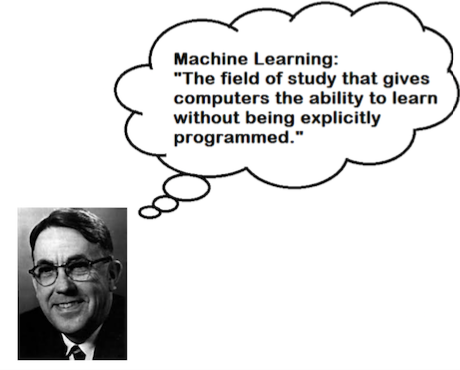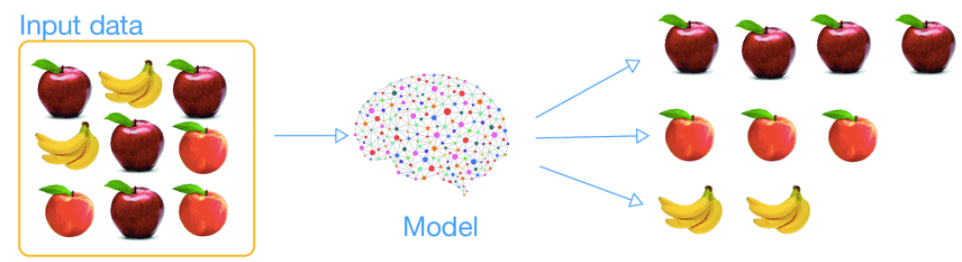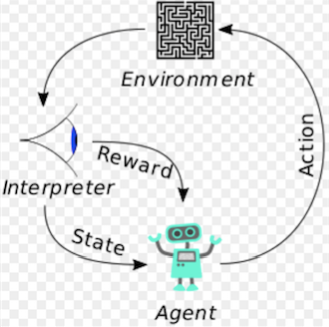# An introduction to Machine LearningPhoto by Alex Knight on Unsplash

As you know, the applications of Machine learning appear everywhere in our life. For example, when you search for something in Google, based on Machine learning algorithms, it recommends to you the most performance results related to your keywords. Facebook, Youtube, Amazon also use recommendation systems for suggesting the users their products. Apple develops Machine learning algorithms for face and fingerprint recognition to activate your devices without using your password, … Thanks to Machine learning, our lives have become much more convenient.

In this article, we will study various types of Machine learning algorithms and their use-cases. The content of this article is divided into 3 parts:

• What is Machine learning?
• Why use Machine learning?
• Types of Machine learning algorithms.

Let’s start!

# I. What is Machine Learning?

In 1959, Arthur Samuel defined: “Machine Learning is the field of study that gives the computer the ability to learn without explicitly programmed”.Definition of Machine learning by Arthur Samuel

In 1997, Tome Michel gave another definition of Machine learning which is more technical: “A computer program is said to learn from experience E with respect to some class of tasks T and performance measure P if its performance at tasks in T, as measured by P, improves with experience E”.

# II. Why use Machine learning?

To see the importance of Machine learning, let consider an example of spam email recognition. For this problem, you first have to examine what a spam email looks like. You remark that it may contain many errors and links, or some words like “bank card”, “free”, “congratulation”, … After analyzing all possible characteristics, you have to write a program to detect them repeatedly for all emails. Therefore, your code will be very long and complicated to maintain. By contrast, the Machine learning technique studies automatically what characteristics are contained in a spam email, the code becomes much shorter and simpler to maintain. Furthermore, machine learning algorithms give us better results compared to the traditional ones.

In summary, there are some reasons why we should use Machine learning algorithms instead of traditional methods:

• Machine learning algorithms are faster, require less computational power, and give better results compared to the traditional algorithms.
• Machine learning techniques can find the solutions for some complicated problems while the traditional methods can not.
• Fluctuating environments: Machine learning systems can adapt to new data.
• Machine learning algorithms are good for the exploration of complicated problems with a large dataset.

Pleases notice that, for building a Machine Learning model, the number of data in the training set must be large enough so that the model can learn correctly. Sometimes, we have to feed the model with thousands of data to get high accuracy. The more various the data, the higher accuracy of the model. That is the reason why the data is so important for Machine Learning and the Data Science domain.

Andrew Ng: “It’s not who has the best algorithms that win. It’s who has the best data”.

According to Forbes, data scientists spend around 80% of their time preparing and managing the data for analysis.

# III. Types of Machine Learning algorithms:

Machine Learning algorithms are classified into 3 principal types:

## 1. Supervised learning

Supervised learning is the most popular algorithm for performing Machine Learning operations. The data, in this case, is labeled. A supervised learning algorithm identifies the features explicitly and carries out prediction or classification accordingly.

a. Classification

The Supervised learning algorithms are applied for the problem of classification when the variables are discrete.

Let’s consider some simple examples for this problem:

Example 1: Spam email detection

We aim to build a Machine Learning model for classifying emails as spam or not spam, based on the number of errors and the number of links in emails. This method is figured out by the following figure. If the number of links and errors of an email is large, then this email will be classified as spam. Otherwise, this email is classified into the non-spam group. We can simply assume that the machine learning model for separating these groups is a straight line.

Once the model is determined, it can be applied for predicting any new email. If the number of errors and links of an email belongs to the lower region of the straight line, then it will be classified as a non-spam email, and vice versa.

Let consider another example of building a Machine learning model for detecting some image objects like fruits.

Example 2: Fruit detection

Given two types of fruits: apples and bananas with their annotations. To build a machine learning model to classify these fruits, the machine will firstly extract some features like color, length, width corresponding to each object. Then, the model is trained on the training set. It learns what features are associated with each label.

After training for the model, we can apply this model for predicting a new sample on the test set which does not have a label.

b. Regression

In the case when the variables are continuous and the target values are not finite, the Machine Learning algorithms can be applied for the regression problem.

Example: House price prediction

Given a dataset of prices of some house in Toulouse, which is figured out in the following figure.

Supposing that the relationship between the house prices and the squares is linear. We aim to build a linear model f(x) = ax+ b that best fits the data. The parameters a and b are estimated so that the following cost function is minimized:

where n denotes the observation number, y_i and f(x_i) denote the true and the predicted value corresponding to the feature x_i, respectively. Once the model is built, it can be applied to predict the price of a new house that did not appear in the given training data.

Some supervised learning algorithms are used popularly as Linear regression, Logistic regression, Support vector machine, Random forest, Gradient Boosting, Artificial neural networks. These algorithms are applied for solving various problems such as image classification, fraud detection, score prediction, …

## 2. Unsupervised learning

Unsupervised learning algorithms are applied in cases where the data is not labeled. This type of algorithm aims to identify the data based on their structures, densities, similar segments, features, ….

Example: Given some groups of fruits without annotations. We aim to build a machine learning model that can classify these fruits based on their colors and their shapes. All fruits which have similar color and shape will be classified to the same group. This type of algorithm is called clustering (or cluster analysis), and it is also a popular technique in Unsupervised learning.The unsupervised algorithm in classifying the data without annotations. (image source: ResearchGate)

There are also some other unsupervised learning algorithms as Principle component analysis, Anomaly detection, Autoencoders, …

Unsupervised learning algorithms are used widely for solving some problems as:

• Customer segmentation for understanding the customers from different groups, to build strategies for marketing.
• Recommender systems, which aim to group all the users with similar patterns to recommend similar contents.
• Anomaly detection, which is applied for fraud detection.

## 3. Reinforcement learning

Reinforcement learning is a machine learning algorithm that allows the machine to learn through interactions with the environment and using feedbacks from its own actions and experiences.

Some elements of Reinforcement learning:

• The environment is the physical world that the agent operates in.
• The state describes the current situation of the agent.
• The reward is the feedback from the environment.
• The policy is the method to map the agent’s state to actions.

The following figure describes briefly how the Reinforcement learning algorithm works. An agent takes actions in an environment, which is interpreted into a reward and the state, then fed back into the agent. This procedure is repeated until the cumulative reward of the agent is maximized.The typical framing of the Reinforcement learning technique (Image source: Wikipedia)

Reinforcement learning is different from Supervised learning. While supervised learning is learning from the training set and its decision is made from the initial input data, the decisions of Reinforcement learning are made sequentially, depending on the input of the current state.

Reinforcement learning is also different from Unsupervised learning. Unsupervised learning aims to find the similarities or the differences between input data, the goal of Reinforcement learning is finding a suitable action model at which the cumulative reward of the agent is maximized.

Reinforcement learning is applied widely in many domains such as robotic manipulation, natural language processing, self-driving cars, industrial automation, gaming, news recommendation, …

In summary, this article introduces briefly Machine learning algorithms as well as their use cases. I hope this helps you to have a quick overview of this domain. If you have any questions, please let me know in the comment. All the contributions to improving this post are welcome.